Question

# The ages of a group of 149 randomly selected adult females have a standard deviation of...

The ages of a group of

149

randomly selected adult females have a standard deviation of

17.5

years. Assume that the ages of female statistics students have less variation than ages of females in the general​ population, so let

sigma

equals17.5years for the sample size calculation. How many female statistics student ages must be obtained in order to estimate the mean age of all female statistics​ students? Assume that we want

95

​%

confidence that the sample mean is within​ one-half year of the population mean. Does it seem reasonable to assume that the ages of female statistics students have less variation than ages of females in the general​ population?

Solution :

Z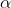/2 = Z0.025 = 1.96

sample size = n = [Z/2*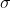/ E] 2

n = [1.96 * 17.5 / 0.5 ]2

n = 4705.96

Sample size = n = 4706

Yes,because statistic students are typically younger than people in the general population.

#### Earn Coins

Coins can be redeemed for fabulous gifts.

Similar Homework Help Questions
• ### The ages of a group of 126 randomly selected adult females have a standard deviation of...

The ages of a group of 126 randomly selected adult females have a standard deviation of 16.7 years. Assume that the ages of female statistics students have less variation than ages of females in the general​ population, so let sigmaequals16.7 years for the sample size calculation. How many female statistics student ages must be obtained in order to estimate the mean age of all female statistics​ students? Assume that we want 90​% confidence that the sample mean is within​ one-half...

• ### The ages of a group of 156156 randomly selected adult females have a standard deviation of...

The ages of a group of 156156 randomly selected adult females have a standard deviation of 16.516.5 years. Assume that the ages of female statistics students have less variation than ages of females in the general​ population, so let sigmaσequals=16.516.5 years for the sample size calculation. How many female statistics student ages must be obtained in order to estimate the mean age of all female statistics​ students? Assume that we want 9898​% confidence that the sample mean is within​ one-half...

• ### The ages of a group of 154 randomly selected adult females have a standard deviation of...

The ages of a group of 154 randomly selected adult females have a standard deviation of 17.6 years. Assume that the ages of female statistics students have less variation than ages of females in the general​ population, so let σ equals=17.6 years for the sample size calculation. How many female statistics student ages must be obtained in order to estimate the mean age of all female statistics​ students? Assume that we want 98​% confidence that the sample mean is within​...

• ### The ages of a group of 160 randomly selected adult females have a standard deviation of...

The ages of a group of 160 randomly selected adult females have a standard deviation of 17.4 years. Assume that the ages of female statistics students have less variation than ages of females in the general​ population, so let sigmaequals17.4 years for the sample size calculation. How many female statistics student ages must be obtained in order to estimate the mean age of all female statistics​ students? Assume that we want 99​% confidence that the sample mean is within​ one-half...

• ### The ages of a group of 122 randomly selected adult females have a standard deviation of...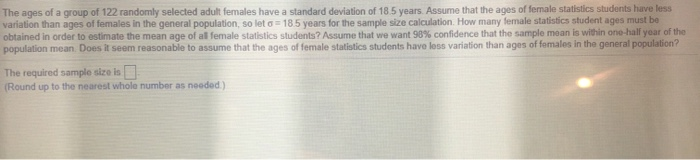The ages of a group of 122 randomly selected adult females have a standard deviation of 18.5 years. Assume that the ages of female statistics students have less variation than ages of females in the general population, so let = 185 years for the sample size calculation. How many female statistics student ages must be obtained in order to estimate the mean age of all female statistics students? Assume that we want 98% confidence that the sample mean is within...

• ### The ages of a group of 152 randomly selected adult females have a standard deviation of...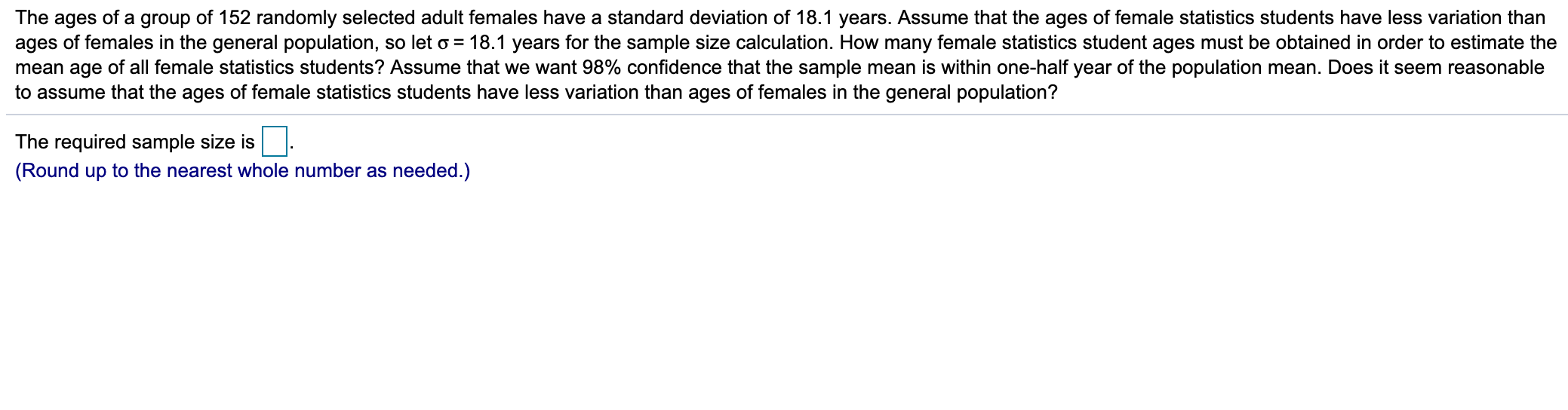The ages of a group of 152 randomly selected adult females have a standard deviation of 18.1 years. Assume that the ages of female statistics students have less variation than ages of females in the general population, so let o = 18.1 years for the sample size calculation. How many female statistics student ages must be obtained in order to estimate the mean age of all female statistics students? Assume that we want 98% confidence that the sample mean is...

• ### The ages of a group of 152 randomly selected adult females have a standard deviation of...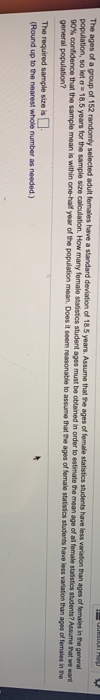The ages of a group of 152 randomly selected adult females have a standard deviation of 18.5 years. Assume that the ages of female statistics students have less variation than ages of females in the general population, so leta 18.5 years for the sample size calculation. How many female statistics student ages must be obtained in order to estimate the mean age of all female statistics students? Assume Pat we want 90% confidence that the sample mean is within one-half...

• ### The ages of a group of 147 randomly selected adult females have a standard deviation of...

The ages of a group of 147 randomly selected adult females have a standard deviation of 18.7 years. Assume that the ages of female statistics students have less variation than ages of females in the general population, so let σ = 18.7 years for the sample size calculation. How many female statistics student ages must be obtained in order to estimate the mean age of all female statistics students? Assume that we want 95% confidence that the sample mean is...

• ### The ages of a group of 142 randomly selected adult females have a standard deviation of...

The ages of a group of 142 randomly selected adult females have a standard deviation of 16.6 years. Assume that the ages of female statistics students have less variation than ages of females in the general​ population, so let sigmaequals16.6 years for the sample size calculation. How many female statistics student ages must be obtained in order to estimate the mean age of all female statistics​ students? Assume that we want 99​% confidence that the sample mean is within​ one-half...

• ### The ages of a group of 121 randomly selected adult females have a standard deviation of...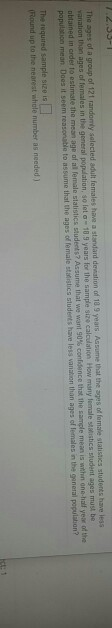The ages of a group of 121 randomly selected adult females have a standard deviation of 10 years Assume that the age of fomate statistics students have less wanation than ages of females in the general population, so leta 10.9 years for the sample size calculation How many female stics student ages must be obtained in order to estimate the mean age of a female sialistics Students Assume that we want 90% confidence that the sample mean is within one-half...